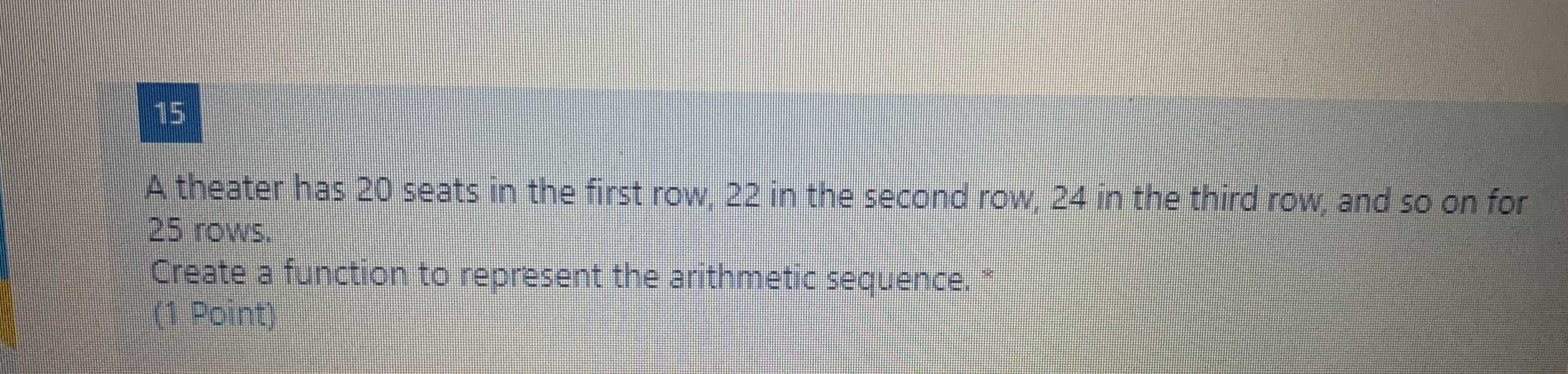### ¿Todavía tienes preguntas de matemáticas?

Pregunte a nuestros tutores expertos
Algebra
Pregunta$$15$$ A theater has $$20$$ seats in the first row, $$22$$ in the second row, $$24$$ in the third row, and so on for

$$25$$ rows. Create a function to represent the arithmetic sequence. (1 Point)

$$t(n) = 18+ 2n$$